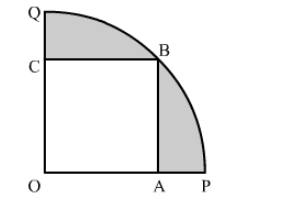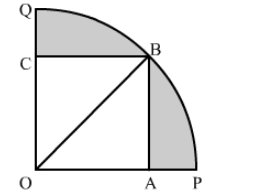# In the given figure, a square OABC is inscribed in a quadrant OPBQ of a circle.`
Question:

In the given figure, a square OABC is inscribed in a quadrant OPBQ of a circle. If OA = 20 cm, find the area of the shaded region. [Use π = 3.14.]Solution:

Construction: Join OBIn right triangle AOB
OB2 = OA2 + AB2
= 202 + 202
= 400 + 400
= 800
∴ OB2 = 800
Area of the shaded region = Area of quadrant OPBQ − Area of Square OABC

$=\frac{1}{4} \pi(\mathrm{OB})^{2}-(\mathrm{OA})^{2}$

$=\frac{1}{4} \times 3.14 \times 800-400$

$=628-400$

$=228 \mathrm{~cm}^{2}$

Hence, the area of the shaded region is 228 cm2.